In Online Education NCERT Solutions for Class 8 Maths Chapter 14 Factorisation Ex 14.2 are part of NCERT Solutions for Class 8 Maths. Here we have given NCERT Solutions for Class 8 Maths Chapter 14 Factorisation Ex 14.2.

 Board CBSE Textbook NCERT Class Class 8 Subject Maths Chapter Chapter 14 Chapter Name Factorisation Exercise Ex 14.2 Number of Questions Solved 5 Category NCERT Solutions

## Online Education NCERT Solutions for Class 8 Maths Chapter 14 Factorisation Ex 14.2

Question 1.
Factorise the following expressions:Solution.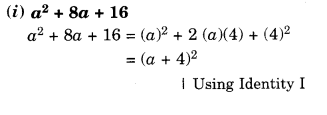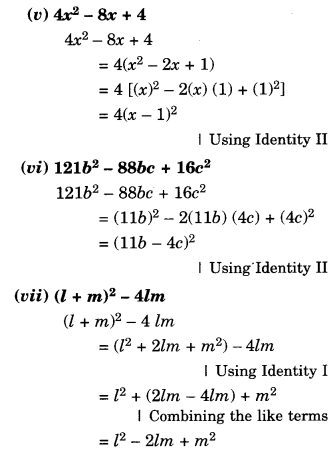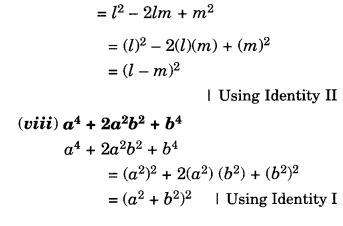Question 2.
Factorise: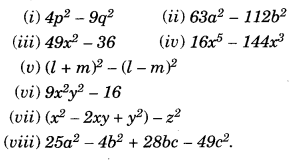Solution.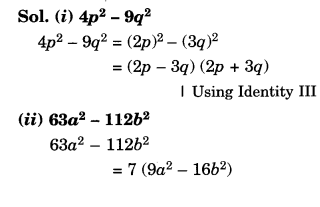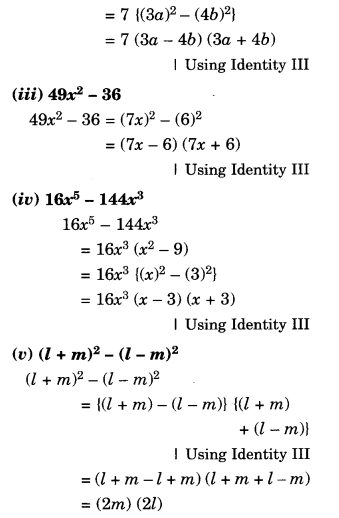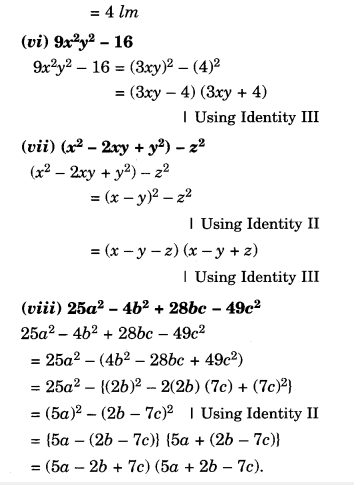Question 3.
Factorise the expressions:Solution.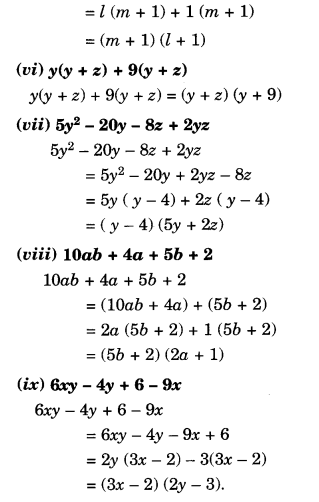Question 4.
Factorise:Solution.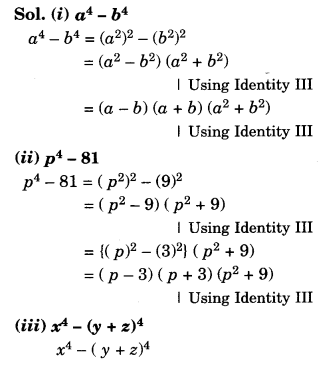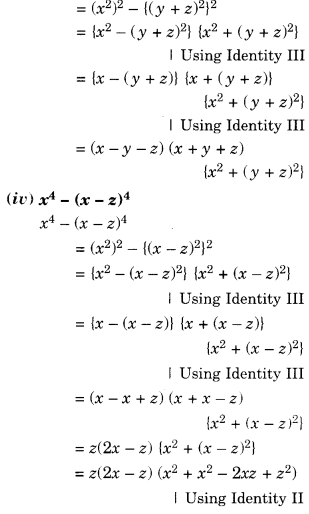Question 5.
Factorise the following expressions: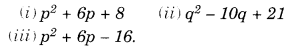Solution.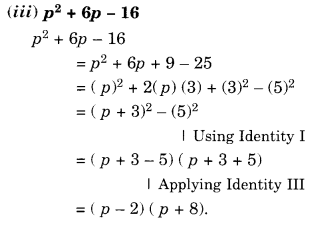We hope the NCERT Solutions for Class 8 Maths Chapter 14 Factorisation Ex 14.2 help you. If you have any query regarding NCERT Solutions for Class 8 Maths Chapter 14 Factorisation Ex 14.2, drop a comment below and we will get back to you at the earliest.